Hooke’s law states that the extension is proportional to the force or tension in a wire if the proportional limit is not exceeded. The constant of proportionality is called modulus of elasticity. Thus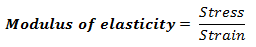Since strain is a pure number, the units of this constant are the same as those of stress, i.e., Nm-2.

### Types of Modulus of Elasticity:

1. Young’s Modulus of Elasticity
2. Bulk Modulus of Elasticity
3. Modulus of Rigidity (η)

## Young’s Modulus

The ratio of tensile (or compressive) stress (σ) to the longitudinal strain (ε) is defined as Young’s modulus and is denoted by the symbol Y.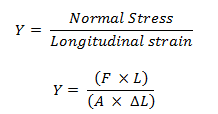Its unit is N/m2 or Pascal and its dimensional formula is [M L-1 T-2].

## Bulk Modulus of Elasticity

The ratio of hydraulic stress to the corresponding hydraulic strain is called bulk modulus.

It is denoted by symbol B.The negative sign indicates the fact that with an increase in pressure, a decrease in volume occurs. That is, if p is positive, ∆V is negative. Thus for a system in equilibrium, the value of bulk modulus B is always positive. SI unit of bulk modulus is the same as that of pressure i.e., Nm-2 or Pa.

The reciprocal of the bulk modulus is called compressibility and is denoted by k. it is defined as the fractional change in volume per unit increase in pressure.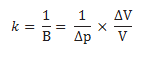The bulk moduli for solids are much larger than for liquids, which are again much larger than the bulk modulus for gases (air).

## Modules of Rigidity (η)

The ratio of shearing stress to the corresponding shearing strain is called the shear modulus of the material. It is also called the modulus of rigidity (?).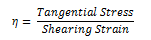Its unit is N/m2 or Pascal and its dimensional formula is [ML-1T-2].

Steel is more elastic than rubber. Solids are more elastic and gases are least elastic.
For liquids, Modulus of rigidity is zero. Young’s modulus (Y) and modulus of rigidity (η) are possessed by solid materials only.

## Poisson’s Ratio

When a wire is loaded, its length increases but its diameter decreases. The strain produced in the direction of applied force is called longitudinal strain and that produced in the perpendicular direction is called lateral strain.

Within the elastic limit, the ratio of lateral strain to the longitudinal strain is called Poisson’s ratio.

Suppose the length of the loaded wire increases from l to l + ∆l and its diameter decreases from D to D – ∆D.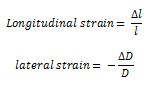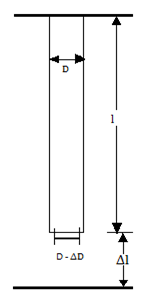Poisson’s ratio

Poisson’s ratio is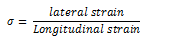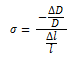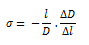The negative sign indicates that longitudinal and lateral strains are in opposite sense.

As the Poisson’s ratio is the ratio of two strains, it has no units and dimensions.

The theoretical value of Poisson’s ratio lies between – 1 and 0.5. Its practical value lies between 0 and 0.5.

### Relation between Y, K, η and σ

• Y = 3K (1 – 2σ)
• Y = 2 η ( 1 + σ)
• σ = 3K – 2η / 2η + 6K
• 9 / Y = 1 / K + 3 / η or Y = 9K η / η + 3K